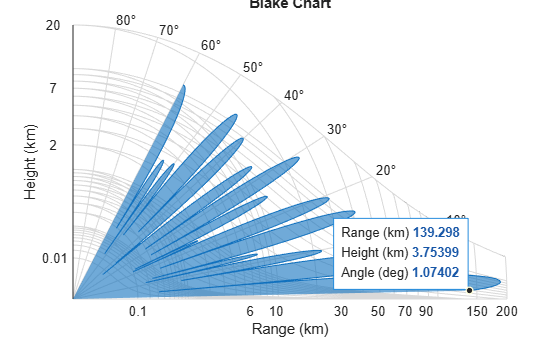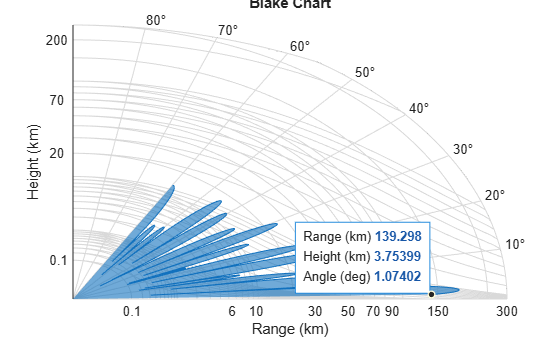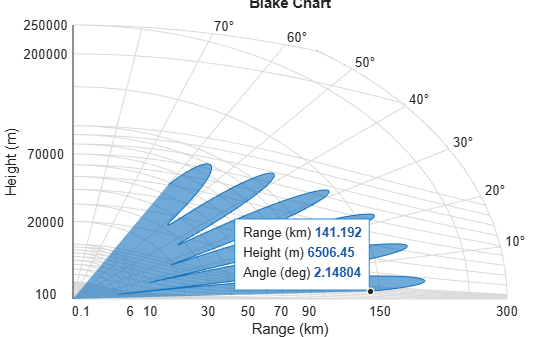# blakechart

Range-angle-height (Blake) chart

## Syntax

``blakechart(vcp,vcpangles)``
``blakechart(vcp,vcpangles,rmax,hmax)``
``blakechart(___,Name,Value)``

## Description

example

````blakechart(vcp,vcpangles)` creates a range-angle-height plot (also called a Blake chart) for a narrowband radar antenna. This chart shows the maximum radar range as a function of target elevation. In addition, the Blake chart displays lines of constant range and lines of constant height. The input consists of the vertical coverage pattern `vcp` and vertical coverage pattern angles `vcpangles`, both produced by `radarvcd`.The range in the range-height-angle chart is the propagated range and the height is relative to the origin of the ray. It is assumed that the antenna height is less than 1000 ft (about 305 meters) above ground level. Normal atmospheric refraction is taken into account using the CRPL Exponential Reference Atmosphere Model. Scattering and ducting are assumed to be negligible.```

example

````blakechart(vcp,vcpangles,rmax,hmax)`, in addition, specifies the maximum range and height of the Blake chart. You can specify range and height units separately in the name-value arguments `RangeUnit` and `HeightUnit`.```

example

````blakechart(___,Name,Value)` allows you to specify additional input parameters using name-value arguments. You can specify multiple name-value arguments in any order with any of the previous syntaxes.```

## Examples

collapse all

Display the vertical coverage diagram of an antenna transmitting at 100 MHz and placed 20 meters above the ground. Set the free-space range to 100 km. Use default plotting parameters.

```freq = 100e6; ant_height = 20; rng_fs = 100; [vcp, vcpangles] = radarvcd(freq,rng_fs,ant_height); blakechart(vcp, vcpangles);```Display the vertical coverage diagram of an antenna transmitting at 100 MHz and placed 20 meters above the ground. Set the free-space range to 100 km. Set the maximum plotting range to 300 km and the maximum plotting height to 250 km.

```freq = 100e6; ant_height = 20; rng_fs = 100; [vcp, vcpangles] = radarvcd(freq,rng_fs,ant_height); rmax = 300; hmax = 250; blakechart(vcp,vcpangles,rmax,hmax)```Plot the range-height-angle curve of a radar having a sinc-function antenna pattern.

Specify antenna pattern

Specify the antenna pattern as a sinc function.

```pat_angles = linspace(-90,90,361)'; pat_u = 1.39157/sind(90/2)*sind(pat_angles); pat = sinc(pat_u/pi);```

Set the transmitting frequency to 100 MHz, the free-space range to 100 km, the antenna tilt angle to ${0}^{\circ }$, and place the antenna 20 meters above the ground. Assume a surface roughness of one meter.

```freq = 100e6; ant_height = 10; rng_fs = 100; tilt_ang = 0; surf_roughness = 1;```

Obtain the vertical coverage pattern values and angles for the radar antenna.

```[vcp, vcpangles] = radarvcd(freq,rng_fs,ant_height,... 'RangeUnit','km','HeightUnit','m',... 'AntennaPattern',pat,... 'PatternAngles',pat_angles,'TiltAngle',tilt_ang,... 'SurfaceHeightStandardDeviation',surf_roughness/(2*sqrt(2)));```

Set the maximum plotting range to 300 km and the maximum plotting height to 250,000 m. Choose the range units as kilometers, `'km'`, and the height units as meters, `'m'`. Set the range and height axes scale powers to 1/2.

```rmax = 300; hmax = 250e3; blakechart(vcp, vcpangles, rmax, hmax, 'RangeUnit','km',... 'ScalePower',1/2,'HeightUnit','m');```## Input Arguments

collapse all

Vertical coverage pattern, specified as a real-valued column vector or matrix. The vertical coverage pattern is the actual maximum range of the radar. Each column of `vcp` corresponds to an individual vertical coverage pattern. Each row of `vcp` corresponds to one of the angles specified in `vcpangles`. Values are expressed in kilometers unless you change the unit of measure using the `RangeUnit` name-value argument.

Example: [282.3831; 291.0502; 299.4252]

Data Types: `double`

Vertical coverage pattern angles, specified as a real-valued column vector. Each element of `vcpangles` specifies the elevation angle in degrees at which a vertical coverage pattern is measured. The set of angles ranges from –90° to 90°.

Example: [2.1480; 2.2340; 2.3199]

Data Types: `double`

Maximum range of plot, specified as a real-valued scalar. Range units are specified by the `'RangeUnit'` name-value argument.

Example: 200

Data Types: `double`

Maximum height of plot, specified as a real-valued scalar. Height units are specified by the `'HeightUnit'` name-value argument.

Example: 100000

Data Types: `double`

### Name-Value Arguments

Specify optional comma-separated pairs of `Name,Value` arguments. `Name` is the argument name and `Value` is the corresponding value. `Name` must appear inside quotes. You can specify several name and value pair arguments in any order as `Name1,Value1,...,NameN,ValueN`.

Example: `'RangeUnit','m'`

Range units denoting nautical miles, miles, kilometers, feet, meters, or kilofeet. This name-value argument specifies the units for the vertical coverage pattern input argument, `vcp`, and the maximum range input argument `rmax`.

Example: `'mi'`

Data Types: `char`

Height units, specified as one of `'nmi'`, `'mi'`, `'km'`, `'ft'`, `'m'`, or `'kft'` denoting nautical miles, miles, kilometers, feet, meters, or kilofeet, respectively. This name-value argument specifies the units for the maximum height `hmax`.

Example: `'m'`

Data Types: `char`

Scale power, specified as a scalar in the range [0, 1]. This argument specifies the range and height axis scale power.

Example: `0.5`

Data Types: `double`

Surface refractivity in N-units, specified as a nonnegative real-valued scalar. The surface refractivity is a parameter of the CRPL Exponential Reference Atmosphere Model used by `blakechart`.

Data Types: `double`

Refraction exponent, specified as a nonnegative real-valued scalar. The refraction exponent is a parameter of the CRPL Exponential Reference Atmosphere Model used by `blakechart`.

Data Types: `double`

Antenna height, specified as a real-valued scalar. When you provide the antenna height, the height in the Blake chart is the height above ground level. Otherwise, the height in the Blake chart is relative to the origin of the ray, and the function assumes that the antenna is less than 1000 ft (about 305 m) above ground level. Use the `HeightUnit` argument to specify the units of `AntennaHeight`.

Data Types: `double`

Face color of vertical coverage pattern patch, specified as a color name, a short name, a hexadecimal color code, an RGB triplet, or `'none'`. If you specify more than one color, the number of colors must match the number of columns of `vcp`.

For a custom color, specify an RGB triplet or a hexadecimal color code.

• An RGB triplet is a three-element row vector whose elements specify the intensities of the red, green, and blue components of the color. The intensities must be in the range `[0,1]`; for example, ```[0.4 0.6 0.7]```.

• A hexadecimal color code is a character vector or a string scalar that starts with a hash symbol (`#`) followed by three or six hexadecimal digits, which can range from `0` to `F`. The values are not case sensitive. Thus, the color codes `'#FF8800'`, `'#ff8800'`, `'#F80'`, and `'#f80'` are equivalent.

Alternatively, you can specify some common colors by name. This table lists the named color options, the equivalent RGB triplets, and hexadecimal color codes.

Color NameShort NameRGB TripletHexadecimal Color CodeAppearance
`'red'``'r'``[1 0 0]``'#FF0000'``'green'``'g'``[0 1 0]``'#00FF00'``'blue'``'b'``[0 0 1]``'#0000FF'``'cyan'` `'c'``[0 1 1]``'#00FFFF'``'magenta'``'m'``[1 0 1]``'#FF00FF'``'yellow'``'y'``[1 1 0]``'#FFFF00'``'black'``'k'``[0 0 0]``'#000000'``'white'``'w'``[1 1 1]``'#FFFFFF'``'none'`Not applicableNot applicableNot applicableNo color

Here are the RGB triplets and hexadecimal color codes for the default colors MATLAB® uses in many types of plots.

`[0 0.4470 0.7410]``'#0072BD'``[0.8500 0.3250 0.0980]``'#D95319'``[0.9290 0.6940 0.1250]``'#EDB120'``[0.4940 0.1840 0.5560]``'#7E2F8E'``[0.4660 0.6740 0.1880]``'#77AC30'``[0.3010 0.7450 0.9330]``'#4DBEEE'``[0.6350 0.0780 0.1840]``'#A2142F'`Example: `'black'`

Example: `'k'`

Example: `[0.850 0.325 0.098]`

Example: `'#D95319'`

Data Types: `double` | `char` | `string`

Edge color of vertical coverage pattern patch, specified as a color name, a short name, a hexadecimal color code, an RGB triplet, or `'none'`. If you specify more than one color, the number of colors must match the number of columns of `vcp`.

For a custom color, specify an RGB triplet or a hexadecimal color code.

• An RGB triplet is a three-element row vector whose elements specify the intensities of the red, green, and blue components of the color. The intensities must be in the range `[0,1]`; for example, ```[0.4 0.6 0.7]```.

• A hexadecimal color code is a character vector or a string scalar that starts with a hash symbol (`#`) followed by three or six hexadecimal digits, which can range from `0` to `F`. The values are not case sensitive. Thus, the color codes `'#FF8800'`, `'#ff8800'`, `'#F80'`, and `'#f80'` are equivalent.

Alternatively, you can specify some common colors by name. This table lists the named color options, the equivalent RGB triplets, and hexadecimal color codes.

Color NameShort NameRGB TripletHexadecimal Color CodeAppearance
`'red'``'r'``[1 0 0]``'#FF0000'``'green'``'g'``[0 1 0]``'#00FF00'``'blue'``'b'``[0 0 1]``'#0000FF'``'cyan'` `'c'``[0 1 1]``'#00FFFF'``'magenta'``'m'``[1 0 1]``'#FF00FF'``'yellow'``'y'``[1 1 0]``'#FFFF00'``'black'``'k'``[0 0 0]``'#000000'``'white'``'w'``[1 1 1]``'#FFFFFF'``'none'`Not applicableNot applicableNot applicableNo color

Here are the RGB triplets and hexadecimal color codes for the default colors MATLAB uses in many types of plots.

`[0 0.4470 0.7410]``'#0072BD'``[0.8500 0.3250 0.0980]``'#D95319'``[0.9290 0.6940 0.1250]``'#EDB120'``[0.4940 0.1840 0.5560]``'#7E2F8E'``[0.4660 0.6740 0.1880]``'#77AC30'``[0.3010 0.7450 0.9330]``'#4DBEEE'``[0.6350 0.0780 0.1840]``'#A2142F'`Example: `'black'`

Example: `'k'`

Example: `[0.850 0.325 0.098]`

Example: `'#D95319'`

Data Types: `double` | `char` | `string`

collapse all

### CRPL Exponential Reference Atmosphere Model

Atmospheric refraction evidences itself as a deviation in an electromagnetic ray from a straight line due to variation in air density as a function of height. The Central Radio Propagation Laboratory (CRPL) exponential reference atmosphere model treats refraction effects by assuming that the index of refraction n(h) and the refractivity N decay exponentially with height. The model defines

`$N=\left(n\left(h\right)-1\right)×{10}^{6}={N}_{\text{s}}{e}^{-{R}_{\text{exp}}h},$`

where Ns is the atmospheric refractivity value (in units of 10–6) at the surface of the earth, Rexp is the decay constant, and h is the height above the surface in kilometers. Thus

`$n\left(h\right)=1+\left({N}_{\text{s}}\text{\hspace{0.17em}}×{10}^{-6}\right){e}^{-{R}_{\text{exp}}h}.$`

The default value of Ns is 313 N-units and can be modified using the `SurfaceRefractivity` name-value argument in functions that accept it. The default value of Rexp is 0.143859 km–1 and can be modified using the `RefractionExponent` name-value argument in functions that accept it.

 Blake, Lamont V. Machine Plotting of Radar Vertical-Plane Coverage Diagrams. Naval Research Laboratory Report 7098, 1970.

 Bean, B.R., and G.D. Thayer. "Central Radio Propagation Laboratory Exponential Reference Atmosphere." Journal of Research of the National Bureau of Standards, Section D: Radio Propagation 63D, no. 3 (November 1959): 315. https://doi.org/10.6028/jres.063D.031.